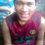# The Buffling Cosines

Prove that $$\cos \frac{\pi}{7} - \cos \frac{2\pi}{7} + \cos \frac{3\pi}{7} = \frac{1}{2}$$

I have done everything i know with this, even using the sum to product identities, still it doesn't work. Please help. thanks.Note by Jaydee Lucero
7 years, 7 months ago

This discussion board is a place to discuss our Daily Challenges and the math and science related to those challenges. Explanations are more than just a solution — they should explain the steps and thinking strategies that you used to obtain the solution. Comments should further the discussion of math and science.

When posting on Brilliant:

• Use the emojis to react to an explanation, whether you're congratulating a job well done , or just really confused .
• Ask specific questions about the challenge or the steps in somebody's explanation. Well-posed questions can add a lot to the discussion, but posting "I don't understand!" doesn't help anyone.
• Try to contribute something new to the discussion, whether it is an extension, generalization or other idea related to the challenge.

MarkdownAppears as
*italics* or _italics_ italics
**bold** or __bold__ bold
- bulleted- list
• bulleted
• list
1. numbered2. list
1. numbered
2. list
Note: you must add a full line of space before and after lists for them to show up correctly
paragraph 1paragraph 2

paragraph 1

paragraph 2

[example link](https://brilliant.org)example link
> This is a quote
This is a quote
    # I indented these lines
# 4 spaces, and now they show
# up as a code block.

print "hello world"
# I indented these lines
# 4 spaces, and now they show
# up as a code block.

print "hello world"
MathAppears as
Remember to wrap math in $$ ... $$ or $ ... $ to ensure proper formatting.
2 \times 3 $2 \times 3$
2^{34} $2^{34}$
a_{i-1} $a_{i-1}$
\frac{2}{3} $\frac{2}{3}$
\sqrt{2} $\sqrt{2}$
\sum_{i=1}^3 $\sum_{i=1}^3$
\sin \theta $\sin \theta$
\boxed{123} $\boxed{123}$

Sort by:

$\cos \frac{ 2\pi }{ 7 }+\cos \frac{ 4\pi }{ 7 }+\cos \frac{ 6\pi }{ 7 }$$\frac{ \sin \frac{ 3\pi }{ 7 }*\cos \frac{ 3\pi }{ 7 } }{ \sin \frac{ \pi }{ 7 } }$$\frac{ \sin \frac{ 6\pi }{ 7 } }{ 2\sin \frac{ \pi }{ 7 } }$$\frac{ 1 }{ 2 }$

- 7 years, 7 months ago

right

- 7 years, 7 months ago

Recall that $\cos(\pi-k)=-\cos k$. This means

$\cos\frac{\pi}{7}-\cos\frac{2\pi}{7}+\cos\frac{3\pi}{7}-\left(\cos\frac{6\pi}{7}-\cos\frac{5\pi}{7}+\cos\frac{4\pi}{7}\right)=1$

Rearranging, we get

$0=-1+\cos\frac{\pi}{7}-\cos\frac{2\pi}{7}+\cos\frac{3\pi}{7}-\cos\frac{4\pi}{7}+\cos\frac{5\pi}{7}-\cos\frac{6\pi}{7}$

Letting $u=e^\frac{i\pi}{7}$, this is

$0=\mathrm{Re}(-1+u-u^2+u^3-u^4+u^5-u^6)$

But, since $u^7-1=(u-1)(u^6-u^5+u^4-u^3+u^2-u+1)=0$, we know either $u=1$ or $u^6-u^5+u^4-u^3+u^2-u+1=0$. Clearly $u\neq1$ since $\mathrm{Re}(u)=\sin\frac{\pi}{7}\neq0$, so

$u^6-u^5+u^4-u^3+u^2-u+1=0$

which completes the proof since $\mathrm{Re}(0)=0$ as desired.

- 7 years, 7 months ago

Since $\cos\frac\pi 7 = -\cos\frac{6\pi}7$ and $\cos\frac{3\pi}7 = -\cos\frac{4\pi}7$, it suffices to prove $\cos\frac{2\pi}7+\cos\frac{4\pi}7+\cos\frac{6\pi}7=-\frac 12$

But, $0=1+\cos\frac{2\pi}7+\cos\frac{4\pi}7+\dots+\cos\frac{12\pi}7=1+2\left(\cos\frac{2\pi}7+\cos\frac{4\pi}7+\cos\frac{6\pi}7\right)$ because $1,\cos\frac{2\pi}7,\cos\frac{4\pi}7,\dots,\cos\frac{12\pi}7$ are the real parts of the 7th roots of unity. The result follows.

- 7 years, 7 months ago

Nice application!

Staff - 7 years, 7 months ago

Thanks, very nicely explained.

- 7 years, 7 months ago

Use the 7th root of unity...I'm sure you will get the answer..

- 7 years, 7 months ago

ill try this one... :D

- 7 years, 7 months ago

How? The cube root of unity would involve $i$ but there is no $i$ in the equation to be proved.

- 7 years, 7 months ago

Recall that $\cos z = \frac{e^{iz} + e^{-iz}}{2}.$ Also, observe that $-\cos \frac{2\pi}{7} = \cos \frac{5\pi}{7}.$ Now let $\zeta_n = e^{2i\pi/n}$ be a primitive $n^{\rm th}$ root of unity. Thus \begin{aligned} \cos \frac{\pi}{7} - \cos \frac{2\pi}{7} + \cos \frac{3\pi}{7} &= \cos \frac{\pi}{7} + \cos \frac{3\pi}{7} + \cos \frac{5\pi}{7} \\ &= \frac{1}{2}(\zeta_{14} + \zeta_{14}^{-1} + \zeta_{14}^3 + \zeta_{14}^{-3} + \zeta_{14}^5 + \zeta_{14}^{-5} ) \\ &= \frac{1}{2}(\zeta_{14} + \zeta_{14}^{13} + \zeta_{14}^3 + \zeta_{14}^{11} + \zeta_{14}^5 + \zeta_{14}^{9} ) \\ &= \frac{1}{2}\left( -\zeta_{14}^7 + \sum_{k=0}^6 \zeta_{14}^{2k+1} \right) \\ &= \frac{1}{2}\left( 1 + \zeta_{14} \sum_{k=0}^6 \zeta_7^k \right) \\ &= \frac{1}{2}(1 + \zeta_{14} \cdot 0) = \frac{1}{2}. \end{aligned} Note that we used the following facts: $\zeta_{2n}^{2k} = \zeta_n^k,$ and because $\{ 1, \zeta_n, \zeta_n^2, \ldots, \zeta_n^{n-1} \}$ are the roots of $z^n - 1 = 0$, the sum of these roots is the negative of the coefficient of the degree $n-1$ term, which is zero for any positive integer $n \ge 2$.

I think that you should be able to tell which solution method I prefer.

- 7 years, 7 months ago

If I recall correctly, the official solution is to consider the expression $\sin \frac{\pi}{7} \left( \cos \frac{\pi}{7} - \cos \frac{2\pi}{7} + \cos \frac{3\pi}{7} \right),$ distributing the sine factor and using the product-to-sum identity $\sin \alpha \cos \beta = \frac{1}{2} \left( \sin (\alpha+\beta) + \sin (\alpha - \beta) \right).$

- 7 years, 7 months ago

wow!!! :D now i know how will I apply the product to sum identity. But, how should I remove the sine factor thereafter?

- 7 years, 7 months ago

After you compute and simplify the expression, you will find it equals $\frac{1}{2} \sin \frac{\pi}{7}$, from which the original identity immediately follows.

- 7 years, 7 months ago

and now I got it!!! haha :D thanks , men...

- 7 years, 7 months ago